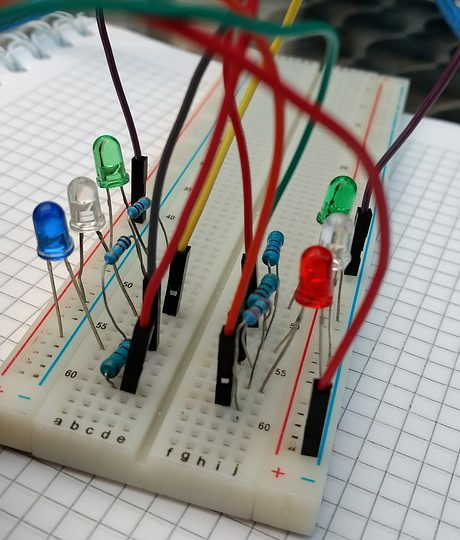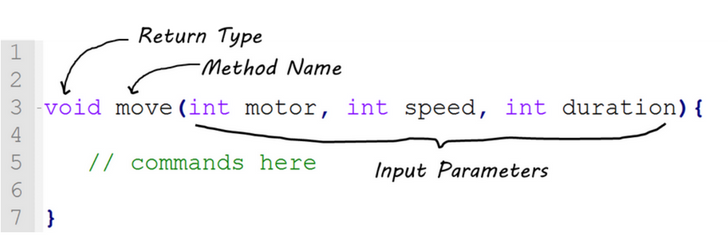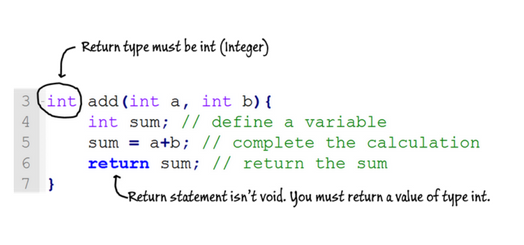Whether you’re a seasoned programmer or new to coding and robotics, writing an Arduino program is fairly easy. In this article, I’ve outlined everything you need to know to get started with Arduino programming.

While not mandatory, I recommend that you have…• Pinterest

## Simple Guide to Arduino Programming (All Steps)

1. Open Arduino IDE (local software or online compiler)
2. File → New
3. Copy and paste the following code into your sketch
```/* Blink
* Written by roboliz
* Beginner Robot Course | learnrobotics.org
*/
//global variables- defined here
int ledPin=13; //built-in LED is on pin 13
//setup- required method for compiling
void setup(){
pinMode(ledPin, OUTPUT); //LEDs are outputs
}
//loop- required method for compiling. runs forever.
void loop(){
digitalWrite(ledPin, HIGH); //turn the led ON
delay(5000); //delay 5000ms or 5sec
digitalWrite(ledPin, LOW); //turn the led OFF
}```
1. Save your program (File → Save)
2. Connect the Arduino to your computer via the USB cord.

You should see the LED blinking on pin 13 of the Arduino Uno!

## Now let’s talk about how the Arduino Program was written

Lines 1-4: Comments used to provide information about the Arduino program. Who wrote the program? What does the program do? When was it written?
Lines 5, 8, 14: Single line comment (use //) used to signify the global variables used in this program. A global variable is an element that can be accessed by any method in a program. Think of methods as functions of code that perform a specific calculation.
Line 6: global variable definition
Line 10: setup method declaration.

## Here’s how to “declare a method”

```// comment - describe the method here
return-type method-name(input-parameters){
// ...code here...
}```

Line 11 Method call: we’re using the built-in method, `pinMode()` to configure the pin that the LED is connected to and signify that it’s an OUTPUT.
Line 16 Loop method declaration (similar to Line 10): `setup()` and `loop()` are required methods for an Arduino program. Therefore you must include both `setup()` and `loop()` in your sketch, or it will not compile.
Line 17, 19 Method call: we’re using the built-in method, `digitalWrite()` to tun the LED ON and OFF (high and low), respectively.
Line 18 Delay the program: The built-in method, `delay()` is used by specifying a duration in milliseconds. The above code will tun the LED on for 5 seconds and then shut it off.

### Method Declaration Example• Pinterest

There are Three Parts to defining a method:

1. Return Type: Are you calculating something that has an answer? Do you want to use this answer later? If so, what’s the data type of that answer? (String, int, char, etc.) If not, use the type, “void.”
2. Method Name: Give your method a name that makes sense. (i.e. if your goal is to “move”, then name the method “`move()`“).
3. Input Parameter(s): This is optional. If you want the method to “take in” values for use in commands or calculations, you can define them within the parentheses. Be sure to include the data type (String, int, char, etc.) before the parameter name. If you don’t have any input parameters, use a set of empty parentheses (i.e. `move()`).

Once you’ve defined your method, you can develop commands and calculations for the method within the method body (See the green comment, above.)

### Return Statements

If the method you’re writing doesn’t return an answer, you will see the return type, void, in the method declaration. However, sometimes we will want to return a value from the method and store it in a variable of a specified type.

Let’s take a look at this simple method for adding two numbers:• Pinterest

First, we want to return the sum of two numbers, so the Return Type will be an Integer (int). The method is called, “add”, for clarity. There are two Input Parameters, “a” and “b”, which are both numbers, so they have the int datatype.

Now that the method is defined, let’s look at the body of the code (between the two curly braces).

```int sum;
sum=a+b;
return sum;```

We first define a local variable, sum, with the data type int. A local variable is defined in the same way as global variables; however, they are only accessible within the method to which they’re defined. So, if we had another method called `average()` and wanted to use int sum from `add()`, we wouldn’t be able to do so. You would have to define another variable, either locally or globally, to use in the `average()` method.

Next, we run our calculation using the two inputs, a and b. Let’s add a + b and store it in sum. Finally we write our return statement and return the sum.

### Alternative Solutions

There are many ways to code a solution to the same problem. Here are a couple more ways to obtain the sum. Let’s talk about each of them.

You could also write the code this way:

```int sum=a+b;
return sum;```

Instead of defining sum on its own line as a local variable that exists without a value, we define sum and set it equal to a plus b. Then we return the sum.

And lastly, this way:

`return a+b;`

Furthermore, you don’t need to define a local variable, sum for such a simple method. You can just add a and b in the return statement, and it will give you a sum. I recommend using this method for shorter methods or functions to consolidate your code.

Make sure you include the last curly brace } or the code will not compile.

### Using our Method = “Method Call”

Great, so we have a sum method, but how do we use it? The act of using a method is known as “calling a method” or “method call”.
You can do this anywhere inside the body of a method by using this syntax:
`method-name(input-parameters);`

So the method call for sum looks like this:
`sum(1,3);`

Notice I substituted two integer values as the input parameters. When the method is run, 1 will be in place of “a” and 3 will be in place of “b”. Therefore our result will be a+b or 1+3. The returned value will be 4.

You can also call a method and if it returns a value, you can store it into a variable:
`int total = sum(1,3);`

In this example, total will equal 4. The datatype of the variable and the return type must be the same, or the code will not compile.
`string total = sum(1,3);`

This would result in a compile error because total is of type String and the result of `sum(1,3)` is of type int.

### The Method “Template” – Reuse this code

If you’ve reviewed the code above, you’ll notice there’s a pattern to writing methods. Here’s a quick template I like to use before writing the method declaration. You can use this for your Arduino Program or any C-based programming language.

Will this method return a value? If so, use int, bool, String, char, etc. If not, use void.
return-type:

What’s the method’s name?
method-name:

Do you need to input anything into the method? If so, use int, bool, String, char, etc. If you have more than one, separate them with a comma. If not, leave the parentheses blank.
input-parameters:

What does this method do? Give it a quick description.
functionality:

Fill out those 4 categories and then you can substitute (copy/paste) them into this format:

```//functionality
return-type method-name(input-parameters){
// ... code goes here ...
}```

Pretty easy, huh?!

### Write your own Arduino Program

In this tutorial, we learned how to define global and local variables, define a method, call a method, and configure Arduino with the required `setup()` and `loop()` methods. You can use the Method Template (above) anytime you want to create a new method. It is good practice to define methods prior to their method call, which means if you wanted to use the `add()` method from above in the `loop()` method, I’d recommend defining it above the `loop()` method declaration.

With this new skillset, let’s do some practice. I’ve provided a sample problem below. Give it a try, and if you have questions, feel free to find me on here in the comments section or on Social Media – Facebook & Instagram (@learnrobotics).

## Practice Problem – Test your knowledge

Going back to the LED problem, let’s make it a little more difficult. 😉

Suppose we have 3 LEDs – 1 red, 1 yellow, and 1 green. (Do you know where we’re going with this…?)

We want to demonstrate a traffic light:

• RED for 30 seconds
• GREEN for 15 seconds
• Blinks YELLOW for 5 seconds
• Yellow for 15 seconds
• This pattern will loops forever.

Create a method called `trafficLight()` that takes in 3 LEDs, using the requirements, above.

First, use the Method Template to define this method, then translate it into an Arduino program.

If you have 3 LEDs and 3 resistors, wire it up, and test it out! If not, check out this free Arduino simulator, and give your solution a try on there.

### Like this post? Share it!

When you have a working solution, don’t forget to post it on Instagram or Facebook (@learnrobotics) and use tags #learnrobotics #trafficlightproblem
I’d love to see what you’re working on!## Get Notified when Registration Begins!

Be the first to know when we open LR Academy! Plus gain FREE robotics tips to your inbox.